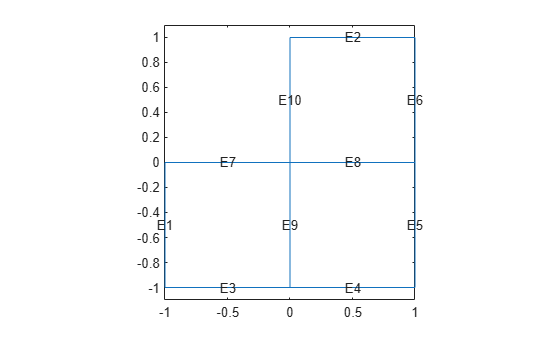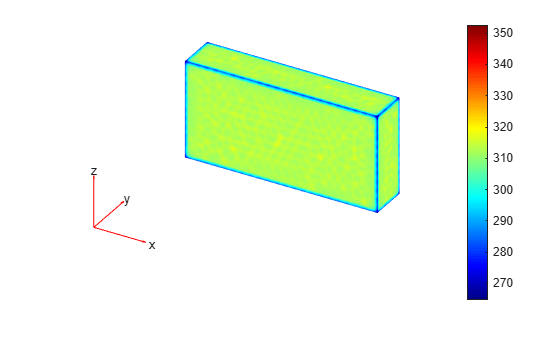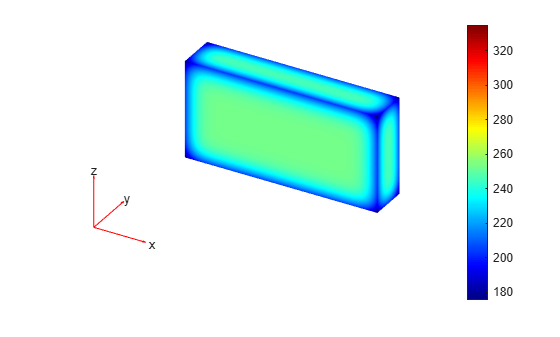# solvepde

Solve PDE specified in a PDEModel

## Syntax

``result = solvepde(model)``
``result = solvepde(model,tlist)``

## Description

example

````result = solvepde(model)` returns the solution to the stationary PDE represented in `model`. A stationary PDE has the property `model.IsTimeDependent = false`. That is, the time-derivative coefficients `m` and `d` in `model.EquationCoefficients` must be `0`.```

example

````result = solvepde(model,tlist)` returns the solution to the time-dependent PDE represented in `model` at the times `tlist`. At least one time-derivative coefficient `m` or `d` in `model.EquationCoefficients` must be nonzero.```

## Examples

collapse all

Create a PDE model, and include the geometry of the L-shaped membrane.

```model = createpde(); geometryFromEdges(model,@lshapeg);```

View the geometry with edge labels.

```pdegplot(model,'EdgeLabels','on') ylim([-1.1,1.1]) axis equal```Set zero Dirichlet conditions on all edges.

`applyBoundaryCondition(model,'dirichlet','Edge',1:model.Geometry.NumEdges,'u',0);`

Poisson's equation is

`$-\nabla \cdot \nabla u=1.$`

Toolbox solvers address equations of the form

`$m\frac{{\partial }^{2}u}{\partial {t}^{2}}+d\frac{\partial u}{\partial t}-\nabla \left(c\nabla u\right)+au=f.$`

Include the coefficients for Poisson's equation in the model.

```specifyCoefficients(model,'m',0,... 'd',0,... 'c',1,... 'a',0,... 'f',1);```

Mesh the model and solve the PDE.

```generateMesh(model,'Hmax',0.25); results = solvepde(model);```

View the solution.

`pdeplot(model,'XYData',results.NodalSolution)`Create a model with 3-D rectangular block geometry.

```model = createpde(); importGeometry(model,'Block.stl');```

Suppose that radiative cooling causes the solution to decrease as the cube of temperature on the surface of the block.

```gfun = @(region,state)-state.u.^3*1e-6; applyBoundaryCondition(model,'neumann','Face',1:model.Geometry.NumFaces,'g',gfun);```

The model coefficients have no source term.

```specifyCoefficients(model,'m',0,... 'd',1,... 'c',1,... 'a',0,... 'f',0);```

The block starts at a constant temperature of 350.

`setInitialConditions(model,350);`

Mesh the geometry and solve the model for times 0 through 20.

```generateMesh(model); tlist = 0:20; results = solvepde(model,tlist);```

Plot the solution on the surface of the block at times 1 and 20.

`pdeplot3D(model,'ColorMapData',results.NodalSolution(:,2))````figure pdeplot3D(model,'ColorMapData',results.NodalSolution(:,21))```## Input Arguments

collapse all

PDE model, specified as a `PDEModel` object. The model contains the geometry, mesh, and problem coefficients.

Example: `model = createpde(1)`

Solution times, specified as a real vector. `tlist` must be a monotone vector (increasing or decreasing).

Example: `0:20`

Data Types: `double`

## Output Arguments

collapse all

PDE results, returned as a `StationaryResults` object or as a `TimeDependentResults` object. The type of `result` depends on whether `model` represents a stationary problem (`model.IsTimeDependent = false`) or a time-dependent problem (`model.IsTimeDependent = true`).

## Tips

• If the Newton iteration does not converge, `solvepde` displays the error message `Too many iterations` or `Stepsize too small`.

• If the initial guess produces matrices containing `NaN` or `Inf` elements, `solvepde` displays the error message ```Unsuitable initial guess U0 (default: U0 = 0)```.

• If you have very small coefficients, or very small geometric dimensions, `solvepde` can fail to converge, or can converge to an incorrect solution. In this case, you might obtain better results by scaling the coefficients or geometry dimensions to be of order one.

### Topics

Introduced in R2016a

## SupportGet trial now